# Category: noncommutative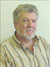Guest post by Fred Van Oystaeyen.

In my book “Virtual Topology and Functorial Geometry” (Taylor and Francis, 2009) I proposed a noncommutative version of space-time ; it is a toy model, but mathematically correct and I included a few philosophical remarks about : “What if reality is noncommutative ?”.

I want to add a few ideas about how “strange” ideas in quantum mechanics all fit naturally in the noncommutative world. First let us talk about noncommutative geometry in an intuitive way.

Then noncommutative space may be thought of as a set of noncommutative places but these noncommutative places need not be sets, in particular they are not sets of points. There is a noncommutative join $\vee$ and a noncommutative intersection $\wedge$, and they satify the axioms (very natural ones) of a noncommutative topology.

The non-commutativity is characterized by the existence of non $\wedge$-idempotent places, i.e. places with a nontrivial self intersection. This allows the $\wedge$ to be noncommutative. From algebraic geometric it follows that one may be interested to let $\vee$ be an abelian operation (hence defining a virtual topology) so let us assume this from hereon.

The set of $\wedge$-idempotent noncommutative-places forms the “commutative shadow” of the noncommutative space; it has operations $\vee$ and $\mathop{\wedge}\limits_{\bullet}$ which are abelian and $\sigma \mathop{\wedge}\limits_{\bullet}\tau$ may be thought of as the largest $\wedge$-idempotent smaller than $\sigma$ and $\tau$ in the partial ordering of the noncommutative space.

The $\wedge$-idempotent noncommutative places are sets in a commutative topology and these are the observable places in the noncommutative space. In the book I present a dynamic (time !) model allowing further elaboration on the noncommutative space but for now let us stick to the intuitive model and assume that space is in fact noncommutative with commutative shadow built upon our space time of physics.

In fact all observations, measurings and predictions made in physics are not about reality but about our observations of reality, so it may be an eternal fact that our observations of reality are described in our brains by commutative methods. Nevertheless we can observe effect of objects existing at noncommutative places in “neighboring” $\wedge$-idempotents sets or observable places.

First if an object exists at a noncommutative place it also exists at all subplaces (a harmless assumption not really essential for the rest). So if there is a noncommutative place, where some object exists, parts of this object may be observed at idempotent subplaces of the noncommutative place. These may even be disjoint in the commutative shadow, not “too far apart” as one object exists on the total noncommutative space.

Since only a part of the noncommutative object is observed on the $\wedge$-idempotent subplace it is not clear that one may actually recognize the observations at different commutative places as belonging to the same noncommutative object. Once one observes one observable place that object seems to exist only on that (commutative) place. Hence a quantum particle can be thought of as existing on several “places” but once observed it looks like it only exists there. This is a first natural phenomenon reflecting “strange” quantum mechanical principles.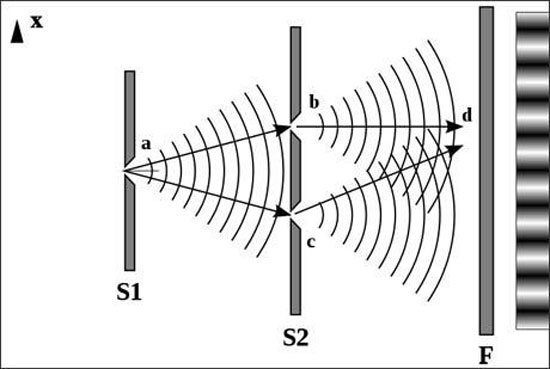Secondly let us look at the double slit experiment. The slits correspond to commutative places $\sigma_1$ and $\sigma_2$ and $\sigma_1 \mathop{\wedge}\limits_{\bullet}\sigma_2=\emptyset$, however in the noncommutative world $\sigma_1\wedge\sigma_2$ need not be empty, only it has no $\wedge$-idempotent subplaces !

Therefore if a photon is defined on a noncommutative place with “light”-effect on observable places “near enough” to it (in a neighborhood small enough to have an observable effect say) then the photon may pass though both slits without splitting or without splitting reality (parallel universes) but just moving into the noncommutative space inside $\sigma_1$ and $\sigma_2$ !

The observable effect at the slits will appear in commutative places near enough (for example, intersecting) to $\sigma_1$ or to $\sigma_2$. As the photon moves on, observable effects will appear in commutative places intersecting the one near to $\sigma_1$ or the one near to $\sigma_2$ and these may themselves have nonempty intersections.

At the moment the effect via $\sigma_1$ interacts with the effect via $\sigma_2$. As the photon progresses in its observed direction other $\wedge$-idempotents showing observable effects may meet and so several interactions between observable effects (via $\sigma_1$ and $\sigma_2$) build a picture of interference.

The symmetry of this picture actually suggests a symmetric arrangement of commutative places around a noncommutative place. So the noncommutativity of space may explain this phenomenon without holographic principle or parallel universes.

In a similar way dark mass may well be mass existing in a non-observable noncommutative place (i.e. containing no observable places). If a lot of mass is in a non-observable noncommutative place its gravity may pull matter from surrounding observable places into the noncommutative place and this may explain black holes. All kinds of problems relating to black holes may have natural non commutative solutions, e.g. information may pass from observable places to noncommutative places and is not lost, only non-observable.

In fact is the definition of information not depending on the nature and capability of the recipient ? There are many philosophically interesting ramifications of these ideas, for example every chemical or neurochemical activity should also be placed in the noncommutative space.

In the book I mentioned how “free will” could be a noncommutative space aspect of the brain activity. I also mention a possible relations with string theory. I am not a specialist in all these things but now I reached the point that I “feel” noncommutative space is a better approximation of the reality and one should investigate it further.

The odd Knight of the round table problem asks for a consistent placement of the n-th Knight in the row at an odd root of unity, compatible with the two different realizations of the algebraic closure of the field with two elements.

The first identifies the multiplicative group of its non-zero elements with the group of all odd complex roots of unity, under complex multiplication. The second uses Conway’s ‘simplicity rules’ in ONAG to define an addition and multiplication on the set of all ordinal numbers.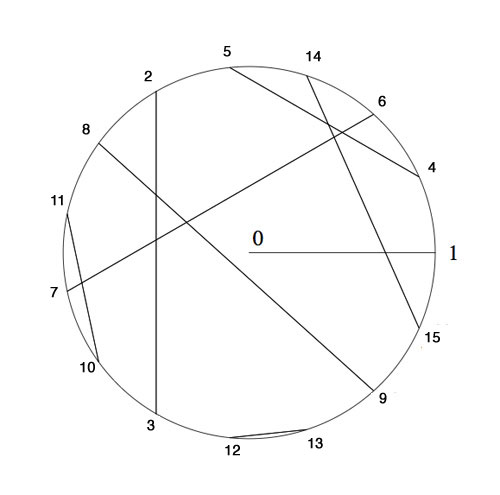Here’s the seating arrangement for the first 15 knights. Conway proved that all finite ordinals smaller than $2^{2^i}$ form a subfield of $\overline{\mathbb{F}}_2$. The first non-trivial one being $\{ 0,1,2,3 \}$ with smallest multiplicative generator $2$, whence we place Knight 2 at $e^{2 i \pi/3}$ and as $2^2=3$ we know where to place the third Knight.

The next subfield is made of the numbers $\{ 0,1,2,\ldots,13,14,15 \}$ and its non-zero numbers form a cyclic group of order 15. Hence we need to find the smallest generator of this group satisfying the additional property of being compatible with the earlier seating, that is, its fifth power must equal to 2. Checking the multiplication table reproduced here one verifies that the wanted generator is 4 and so we place Knight 4 at $e^{\frac{2 \pi i}{15}}$ and as all the ordinals smaller than 16 are powers of 4 this tells us where to place the Knights until we get to the 15th in the row.

In february we were able to seat the first few thousand Knights by showing by hand that 32 is the smallest ordinal such that its 15-th power is equal to 4 and using SAGE that 1051 is the smallest ordinal whose 257-th power equals 32. These calculations enabled us to seat the Knights until we get to the 65536-th in the row.

Today I managed to show that 1361923 is the smallest ordinal such that its 65537-th power equals 1051. You can verify this statement in SAGE using the method explained in the february post

sage: R.< x,y,z,t,u >=GF(2)[]

sage: S.< a,b,c,d,e > =
R.quotient((x^2+x+1,y^2+y+x,z^2+z+x*y,t^2+t+x*y*z,u^2+u+x*y*z*t))

sage: (c*e+b*e+a*b*c*d+b*c*d+a*b*d+a+1)^65537
c^2 + b*d + a + 1

(It takes a bit longer to check minimality of 1361923). That is, we have to seat Knight 1361923 at $e^{\frac{2 \pi i}{4294967295}}$ and because all the numbers smaller than 4294967296 are powers of 1361923 we have seating arrangements for the first 4294967295 Knights!

I did try the same method in february but ran into time- and memory-problems on my 2.4Ghz 2Gb MacBook. Today I upgraded from Sage 3.3 to Sage 4.6 and this version is a lot faster (using the 64-bit architecture) and also appears to be much better at memory-management. Thank you, Sage-community!

Wishing you all a lot of mathematical (and other) fun in the prime-number year 2011.

atb :: lieven.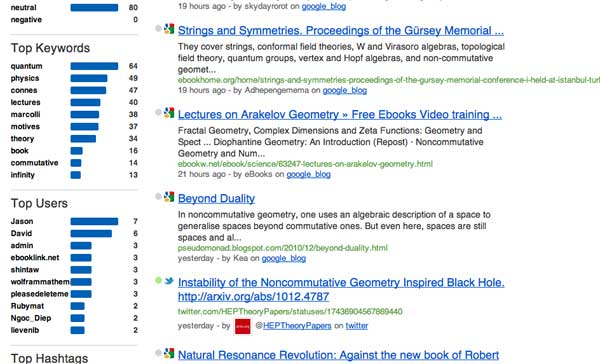SocialMention gives a rather accurate picture of the web-buzz on a specific topic. For this reason I check it irregularly to know what’s going on in noncommutative geometry, at least web-wise.

Yesterday, I noticed two new kids on the block : Jason and David. Their blogs have (so far ) 44 resp. 27 posts, this month alone. My first reaction was: respect!, until I glanced at their content…

David of E-Infinity

Noncommutative geometry has a deplorable track record when it comes to personality-cults and making extra-ordinary claims, but this site beats everything I’ve seen before. Its main mission is to spread the gospel according to E.N.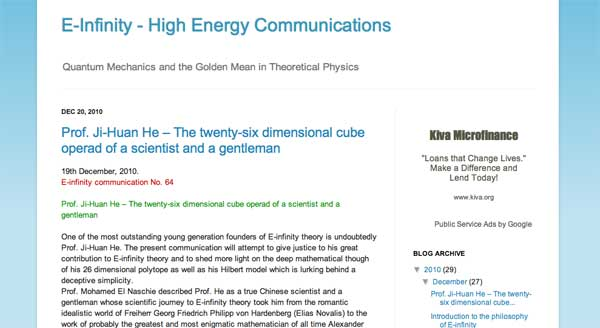A characteristic quote :

“It was no doubt the intention of those well known internet thugs and parasites to distract us from science and derail us from our road. This was the brief given to them by you know who. Never the less we will attempt to give here what can only amount to a summary of the summary of what E. N. considers to be the philosophical background to his theory.”

Jason of the E.N. watch

The blog’s mission statement is to expose the said prophet E.N. as a charlatan.The language used brings us back to the good(?!) old string-war days.

“This is amusing because E. N.’s sockpuppets go on and on about E. N. being a genius polymath with an expert grasp of science, art, history, philosophy and politics. E. N. Watch readers of course know that his knowledge in all areas comes primarily from mass-market popularizations.”

As long as the Connes support-blog and the Rosenberg support-blog remain silent and the Jasons and Davids of this world run the online ncg-show, it is probably better to drop the topic here.

Never a dull moment with Books Ngram Viewer. Pick your favorite topic(s) and try to explain and name valleys and peaks in the Ngram.

An example. I wanted to compare the relative impact of a couple of topics I love, algebraic geometry (blue), category theory (red), representation theory (green) and noncommutative geometry (the bit of yellow in the lower right hand corner…) from 1960 onwards.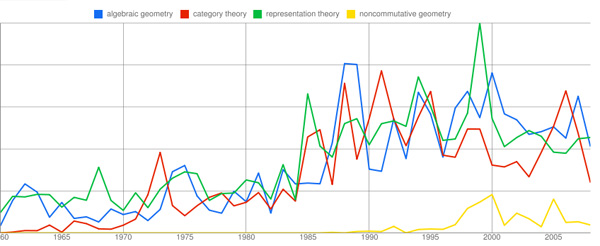I was surprised to find out that the first three topics were almost in the same impact-league, but then Ngram-viewing can be cruel when you’re biased …

Anyone having an explanation/name for the great depressions of 1982, 1993 and 1996?

On the positive side, what happened in 1988-89 or what caused the representation-peak in 1999, or the category-delirium in 2006?

So far, I’ve only been able to pinpoint a couple of events. My favorite being the red peak in 1973, which I’d like to christen “Grothendieck’s folly”.

This is a belated response to a Math-Overflow exchange between Thomas Riepe and Chandan Singh Dalawat asking for a possible connection between Connes’ noncommutative geometry approach to the Riemann hypothesis and the Langlands program.

Here’s the punchline : a large chunk of the Connes-Marcolli book Noncommutative Geometry, Quantum Fields and Motives can be read as an exploration of the noncommutative boundary to the Langlands program (at least for $GL_1$ and $GL_2$ over the rationals $\mathbb{Q}$).

Recall that Langlands for $GL_1$ over the rationals is the correspondence, given by the Artin reciprocity law, between on the one hand the abelianized absolute Galois group

$Gal(\overline{\mathbb{Q}}/\mathbb{Q})^{ab} = Gal(\mathbb{Q}(\mu_{\infty})/\mathbb{Q}) \simeq \hat{\mathbb{Z}}^*$

and on the other hand the connected components of the idele classes

$\mathbb{A}^{\ast}_{\mathbb{Q}}/\mathbb{Q}^{\ast} = \mathbb{R}^{\ast}_{+} \times \hat{\mathbb{Z}}^{\ast}$

The locally compact Abelian group of idele classes can be viewed as the nice locus of the horrible quotient space of adele classes $\mathbb{A}_{\mathbb{Q}}/\mathbb{Q}^{\ast}$. There is a well-defined map

$\mathbb{A}_{\mathbb{Q}}’/\mathbb{Q}^{\ast} \rightarrow \mathbb{R}_{+} \qquad (x_{\infty},x_2,x_3,\ldots) \mapsto | x_{\infty} | \prod | x_p |_p$

from the subset $\mathbb{A}_{\mathbb{Q}}’$ consisting of adeles of which almost all terms belong to $\mathbb{Z}_p^{\ast}$. The inverse image of this map over $\mathbb{R}_+^{\ast}$ are precisely the idele classes $\mathbb{A}^{\ast}_{\mathbb{Q}}/\mathbb{Q}^{\ast}$. In this way one can view the adele classes as a closure, or ‘compactification’, of the idele classes.

This is somewhat reminiscent of extending the nice action of the modular group on the upper-half plane to its badly behaved action on the boundary as in the Manin-Marcolli cave post.

The topological properties of the fiber over zero, and indeed of the total space of adele classes, are horrible in the sense that the discrete group $\mathbb{Q}^*$ acts ergodically on it, due to the irrationality of $log(p_1)/log(p_2)$ for primes $p_i$. All this is explained well (in the semi-local case, that is using $\mathbb{A}_Q’$ above) in the Connes-Marcolli book (section 2.7).

In much the same spirit as non-free actions of reductive groups on algebraic varieties are best handled using stacks, such ergodic actions are best handled by the tools of noncommutative geometry. That is, one tries to get at the geometry of $\mathbb{A}_{\mathbb{Q}}/\mathbb{Q}^{\ast}$ by studying an associated non-commutative algebra, the skew-ring extension of the group-ring of the adeles by the action of $\mathbb{Q}^*$ on it. This algebra is known to be Morita equivalent to the Bost-Connes algebra which is the algebra featuring in Connes’ approach to the Riemann hypothesis.

It shouldn’t thus come as a major surprise that one is able to recover the other side of the Langlands correspondence, that is the Galois group $Gal(\mathbb{Q}(\mu_{\infty})/\mathbb{Q})$, from the Bost-Connes algebra as the symmetries of certain states.

In a similar vein one can read the Connes-Marcolli $GL_2$-system (section 3.7 of their book) as an exploration of the noncommutative closure of the Langlands-space $GL_2(\mathbb{A}_{\mathbb{Q}})/GL_2(\mathbb{Q})$.

At the moment I’m running a master-seminar noncommutative geometry trying to explain this connection in detail. But, we’re still in the early phases, struggling with the topology of ideles and adeles, reciprocity laws, L-functions and the lot. Still, if someone is interested I might attempt to post some lecture notes here.